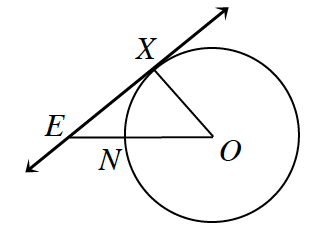### Home > GC > Chapter 10 > Lesson 10.1.5 > Problem10-52

10-52.In the figure at right, $\overleftrightarrow{ E X }$ is tangent to $⊙O$ at point $X$. $OE = 20 \; \text{cm}$ and $XE = 15 \; \text{cm}$.

1. What is the area of the circle?

According to the definition of the tangent line, what is the measure of $∠EXO$?
How can you use this to find the length of $XO$?

A tangent line is perpendicular to a radius, so the measure of $∠EXO = 90º$, so the triangle is a right triangle. So we can use the Pythagorean Theorem.

$\begin{array}{l} \left(XE\right)^2 + \left(XO\right)^2 = \left(OE\right)^2 \\ 15^2 + \left(XO\right)^2 = 20^2 \\ 225 + XO^2 = 400 \\ XO^2 = 175 \\ XO=13.23 \end{array}$

$A = \pi r^2\\ A=\pi (13.23)^2\\ A = 175\pi\text{ cm}^2\\ A = 549.78\text{ cm}^2$

1. What is the area of the sector bounded by $\overline{OX}$ and $\overline{ON}$?

Find the measure of $∠XON$. Use this to find the area of the sector.

$A\approx74.21\text{ cm}^2$

2. Find the area of the region bounded by $\overline{XE}$, $\overline{NE}$, and $\overarc{ N X }$.

Find the area of the triangle formed by $E, X,\text{ and }O$. Subtract the area of the sector from the area of the triangle.